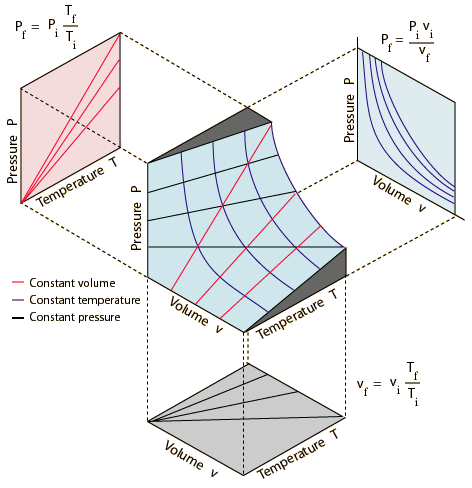Question #8ea79

Mar 17, 2015

You have three variables, $P$, $V$, and $T$, so you need a three-dimensional graph with $P$, $V$, and $T$ axes.

You get a 3D surface that looks like the middle graph in the image below.If the temperature is constant and you look at the graph in a $P V$ plane, you see the blue line hyperbola of Boyle's Law, $P V = k$.

If the pressure is constant and you look at the graph in a $V T$ plane, you see the straight black line of Charles' Law, $V = k T$.

If the volume is constant and you look at the graph in a $P T$ plane, you see the straight red line of Amontons' Law (often called Gay-Lussac's Law), $P = k T$.

Put them all together, and the 3D surface is a graph of the Combined Gas Law, $\frac{P V}{T} = k$.